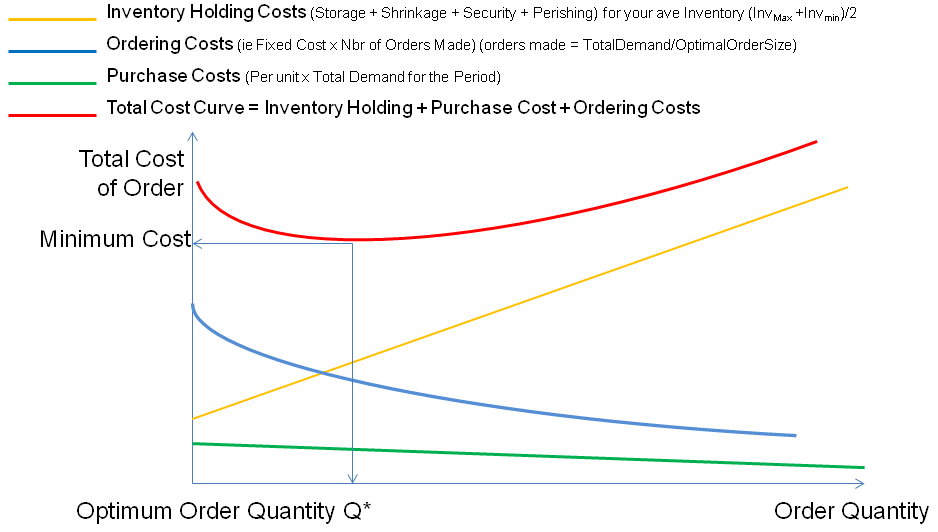# Ordering the right amount of stock

## Optimal Ordering Quantities

This is just a very basic introduction, to a subject that can get very complicated. Nonetheless we illustrate here the basics of ordering stock and inventory and why it requires some thought and you should use this as an example of why communication between parties in a business is so vital!

### Economic order quantity (EOQ)

To research more on this look at production scheduling literature in operations management. The gurus on this are Wilson and Adler. The problem is to order a quantity of stock that minimizes the total holding costs and ordering costs.

#### Overview

To keep things simple we start off with this model

• EOQ applies only when demand for a product is relatively constant over a period say a year
• and each new order is delivered in full when inventory reaches zero. (a minor tweak sorts this out)
• There is a fixed cost for each order placed, regardless of the number of units ordered.
• There is also a cost for each unit held in storage, commonly known as inventory holding cost, usually expressed as a percentage of the purchase cost of the item.
• We want to determine the optimal number of units to order so that we minimize the total cost associated with the purchase, delivery and storage of the product.

#### The Calculation Inputs

What you need to know for the calculation is as follows:

• Your firm's total demand for the period,
• the purchase cost for each item,
• the fixed cost to place the order and
• the storage cost for each item per year.

#### The Calculation Variables

These are the variables you will assign to the inputs listed above

• P = purchase unit price, unit production cost
• Q = any order quantity
• Q* = optimal order quantity
• D = annual demand quantity
• T = fixed total cost per order, setup cost (not per unit, typically cost of ordering and shipping and handling. This is not the cost of goods)
• H = annual holding cost per unit, also known as carrying cost or storage cost (capital cost, warehouse space, refrigeration, insurance, etc. usually not related to the unit production cost)
• C= Cost of the order to your firm

referring to the general graph shown below, all we are doing is finding the best trade-off between ordering cost (blue) and holding cost (yellow) and Purchase cost (green). Total cost (red) You will see that the Purchase cost is not a relevant cost for determining the optimal order quantity!$TotalCost = PurchaseCost + OrderingCost + InventoryHoldingCost$

• $PurchaseCost =$per Unit Cost x Total Amount in Demand $= P × D$
• $OrderingCost$ = Cost of placing an order:(let's call this T) x number of orders made in the period $= T × D/Q$
• $InventoryHoldingCost$ = the average quantity in stock (between full inventory and empty) is $(Q+0)/2$, so this cost is $H × Q/2$

This gives a Total Cost Equation of $C = PD + {\frac{DT}{Q}} + {\frac{HQ}{2}}$

To determine the minimum point of the total cost curve, we need to see at what point the tangent of the curve is zero. To do this we calculate the derivative of the total cost with respect to Q i.e. the change in Cost with respect to the change in Quantity.

And so we get this equation to solve ${0} = -{\frac{DT}{Q^2}}+{\frac{H}{2}}$

Solving for Q gives Q* (the optimal order quantity):$Q^{*2}={\frac{2DT}{H}}$ Thus $Q^* = \sqrt{\frac{2DT}{H}}$

Notice that Q* is independent of P; it is a function of only T, D, H.

CAREFULLY NOTE: We assumed our Minimum inventory was zero, this is probably not realistic, and also we assumed no bulk discounts. Just plug your changes into the overall formula and e-mail us if you can't derive the function to work out your maxima and minima

### Let's try an Example

Plug in some numbers to see how it all hangs together. Let's assume

1. your financial modelling and forecasting indicates a total demand for your product of quantity (D) = 100,000 units
2. the Cost for making an order is order (T) = ZAR 0.5 per unit
3. Your Cost Holding Inventory expressed as a percentage is 5% of the Unit Cost
4. The Unit cost P is ZAR 32
5. You get no bulk order discounts

Thus we get: H = 32 * 0.05 so the Period Inventory Holding cost per unit H = ZAR 1.6

The optimal order quantity is $Q* = \sqrt{\frac{2D*K}{h}} = \sqrt{\frac{2*100,000*0.5}{32*0.05}} =250 units$

Number of orders per year $= {\frac{100,000}{250}} = 400$

The total cost of these orders to your firm is $= P*D + K (D/Q*) + H (Q*/2)$

Giving you an amount $= 8*100,000 + 2 (100,000/250) +1.6 (250/2) = ZAR 801,000$

Check for yourself that any number higher or lower than Q* will cost more.

### What's the message?

Play around with the basic example provided here to see how much it can cost you to get your order quantity wrong. See this as an example of why communication throughout a business is so vital. If your facilities manager negotiates a better rental for storage space, you waste a big opportunity by not letting your purchasing department know!!